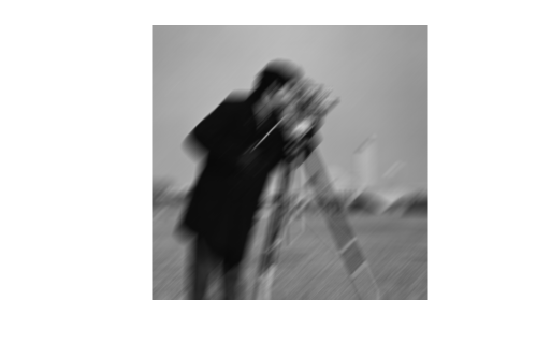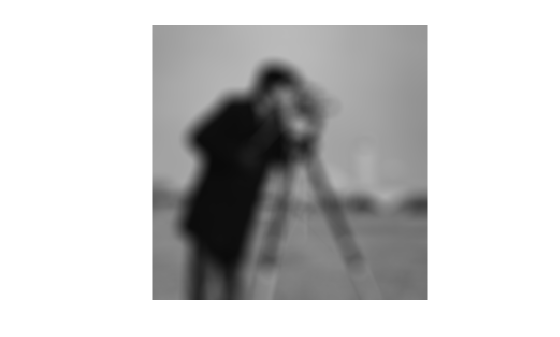# fspecial

Create predefined 2-D filter

## Syntax

``h = fspecial(type)``
``h = fspecial('average',hsize)``
``h = fspecial('disk',radius)``
``h = fspecial('gaussian',hsize,sigma)``
``h = fspecial('laplacian',alpha)``
``h = fspecial('log',hsize,sigma)``
``h = fspecial('motion',len,theta)``
``h = fspecial('prewitt')``
``h = fspecial('sobel')``

## Description

example

````h = fspecial(type)` creates a two-dimensional filter `h` of the specified `type`. Some of the filter types have optional additional parameters, shown in the following syntaxes. `fspecial` returns `h` as a correlation kernel, which is the appropriate form to use with `imfilter`.```
````h = fspecial('average',hsize)` returns an averaging filter `h` of size `hsize`.```
````h = fspecial('disk',radius)` returns a circular averaging filter (pillbox) within the square matrix of size `2*radius+1`.```
````h = fspecial('gaussian',hsize,sigma)` returns a rotationally symmetric Gaussian lowpass filter of size `hsize` with standard deviation `sigma`. Not recommended. Use `imgaussfilt` or `imgaussfilt3` instead.```
````h = fspecial('laplacian',alpha)` returns a 3-by-3 filter approximating the shape of the two-dimensional Laplacian operator, `alpha` controls the shape of the Laplacian.```
````h = fspecial('log',hsize,sigma)` returns a rotationally symmetric Laplacian of Gaussian filter of size `hsize` with standard deviation `sigma`. ```
````h = fspecial('motion',len,theta)` returns a filter to approximate, once convolved with an image, the linear motion of a camera. `len` specifies the length of the motion and `theta` specifies the angle of motion in degrees in a counter-clockwise direction. The filter becomes a vector for horizontal and vertical motions. The default `len` is `9` and the default `theta` is `0`, which corresponds to a horizontal motion of nine pixels.```
````h = fspecial('prewitt')` returns a 3-by-3 filter that emphasizes horizontal edges by approximating a vertical gradient. To emphasize vertical edges, transpose the filter `h'`. [ 1 1 1 0 0 0 -1 -1 -1 ] ```
````h = fspecial('sobel')` returns a 3-by-3 filter that emphasizes horizontal edges using the smoothing effect by approximating a vertical gradient. To emphasize vertical edges, transpose the filter `h'`. [ 1 2 1 0 0 0 -1 -2 -1 ] ```

## Examples

collapse all

```I = imread('cameraman.tif'); imshow(I);```Create a motion filter and use it to blur the image. Display the blurred image.

```H = fspecial('motion',20,45); MotionBlur = imfilter(I,H,'replicate'); imshow(MotionBlur);```Create a disk filter and use it to blur the image. Display the blurred image.

```H = fspecial('disk',10); blurred = imfilter(I,H,'replicate'); imshow(blurred);```## Input Arguments

collapse all

Type of filter, specified as one of the following values:

Value

Description

`'average'`

Averaging filter

`'disk'`

Circular averaging filter (pillbox)

`'gaussian'`

Gaussian lowpass filter. Not recommended. Use `imgaussfilt` or `imgaussfilt3` instead.

`'laplacian'`

Approximates the two-dimensional Laplacian operator

`'log'`

Laplacian of Gaussian filter

`'motion'`

Approximates the linear motion of a camera

`'prewitt'`

Prewitt horizontal edge-emphasizing filter

`'sobel'`

Sobel horizontal edge-emphasizing filter

Data Types: `char` | `string`

Size of the filter, specified as a positive integer or 2-element vector of positive integers. Use a vector to specify the number of rows and columns in `h`. If you specify a scalar, then `h` is a square matrix.

When used with the `'average'` filter type, the default filter size is `[3 3]`. When used with the Laplacian of Gaussian (`'log'`) filter type, the default filter size is `[5 5]`.

Data Types: `double`

Radius of a disk-shaped filter, specified as a positive number. The filter is a square matrix of size `2*radius+1`.

Data Types: `double`

Standard deviation, specified as a positive number.

Data Types: `double`

Shape of the Laplacian, specified as a number in the range [0, 1]. Specify `alpha` as `0` to obtain a 4-neighborhood Laplacian filter:

```[ 0 1 0 1 -4 1 0 1 0 ]```

Data Types: `double`

Linear motion of camera, specified as a numeric scalar, measured in pixels.

Data Types: `double`

Angle of camera motion in degrees, specified as a numeric scalar. The angle is measured in a counter-clockwise direction from horizontal.

Data Types: `double`

## Output Arguments

collapse all

Correlation kernel, returned as a matrix.

Data Types: `double`

## Algorithms

Averaging filters:

```ones(n(1),n(2))/(n(1)*n(2)) ```

Gaussian filters:

`${h}_{g}\left({n}_{1},{n}_{2}\right)={e}^{\frac{-\left({n}_{1}^{2}+{n}_{2}^{2}\right)}{2{\sigma }^{2}}}$`

`$h\left({n}_{1},{n}_{2}\right)=\frac{{h}_{g}\left({n}_{1},{n}_{2}\right)}{\sum _{{n}_{1}}\sum _{{n}_{2}}{h}_{g}}$`

Laplacian filters:

`${\nabla }^{2}=\frac{{\partial }^{2}}{\partial {x}^{2}}+\frac{{\partial }^{2}}{\partial {y}^{2}}$`
`${\nabla }^{2}=\frac{4}{\left(\alpha +1\right)}\left[\begin{array}{ccc}\frac{\alpha }{4}& \frac{1-\alpha }{4}& \frac{\alpha }{4}\\ \frac{1-\alpha }{4}& -1& \frac{1-\alpha }{4}\\ \frac{\alpha }{4}& \frac{1-\alpha }{4}& \frac{\alpha }{4}\end{array}\right]$`

Laplacian of Gaussian (LoG) filters:

`${h}_{g}\left({n}_{1},{n}_{2}\right)={e}^{\frac{-\left({n}_{1}^{2}+{n}_{2}^{2}\right)}{2{\sigma }^{2}}}$`
`$h\left({n}_{1},{n}_{2}\right)=\frac{\left({n}_{1}^{2}+{n}_{2}^{2}-2{\sigma }^{2}\right){h}_{g}\left({n}_{1},{n}_{2}\right)}{{\sigma }^{4}\sum _{{n}_{1}}\sum _{{n}_{2}}{h}_{g}}$`

Note that `fspecial` shifts the equation to ensure that the sum of all elements of the kernel is zero (similar to the Laplace kernel) so that the convolution result of homogeneous regions is always zero.

Motion filters:

1. Construct an ideal line segment with the length and angle specified by the arguments `len` and `theta`, centered at the center coefficient of `h`.

2. For each coefficient location `(i,j)`, compute the nearest distance between that location and the ideal line segment.

3. `h = max(1 - nearest_distance,0);`

4. Normalize `h`: `h = h/(sum(h(:)))`

## Version History

Introduced before R2006a